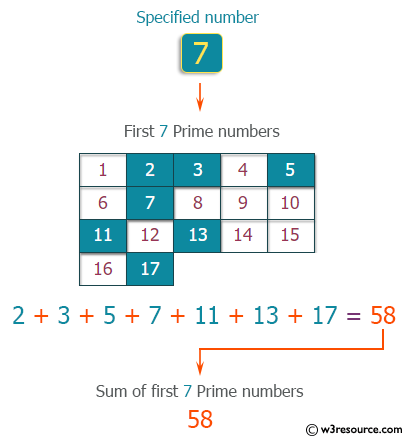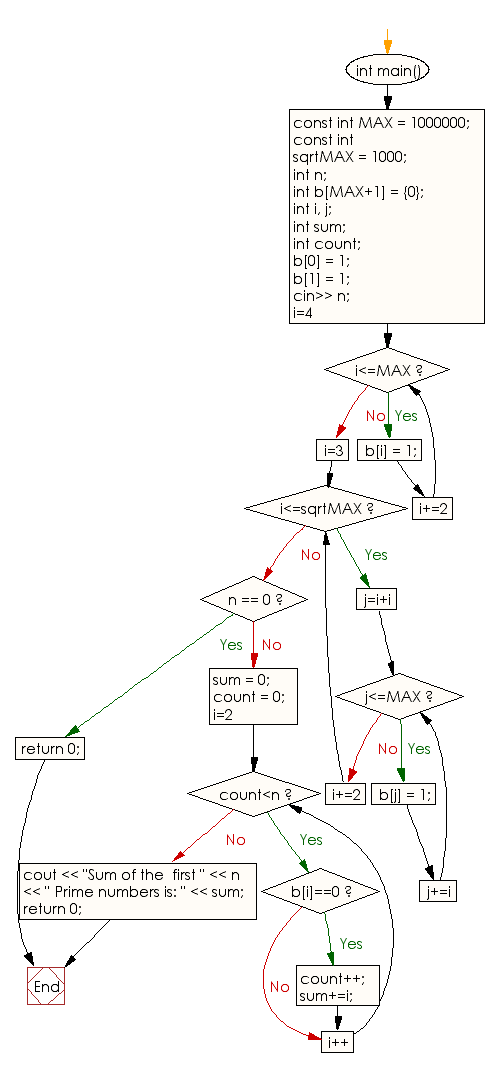﻿ C++ : Compute the sum of the given number of Prime numbers

# C++ Exercises: Compute the sum of the specified number of Prime numbers

## C++ Basic: Exercise-75 with Solution

Write a C++ program to compute the sum of the specified number of prime numbers.

For example when n = 7,
s = 2 + 3 + 5 + 7 + 11 + 13 + 17 = 58.

Pictorial Presentation:Sample Solution:

C++ Code :

``````#include <iostream>
using namespace std;
int main()
{
const int MAX = 1000000;
const int sqrtMAX = 1000;
int n;
int b[MAX+1] = {0};
int i, j;
int sum;
int count;
b = 1;
b = 1;
cin>> n;
for(i=4; i<=MAX; i+=2)
b[i] = 1;
for(i=3; i<=sqrtMAX; i+=2)
for(j=i+i; j<=MAX; j+=i)
b[j] = 1;

if(n == 0)
return 0;
sum = 0;
count = 0;
for(i=2; count<n; i++) {
if(b[i]==0) {
count++;
sum+=i;
}
}
cout << "Sum of the  first " << n << " Prime numbers is: " << sum;
return 0;
}
``````

Sample Output:

```Sample Input: 7
Sum of the  first 7 Prime numbers is: 58
```

Flowchart:C++ Code Editor:

What is the difficulty level of this exercise?

﻿

## C++ Programming: Tips of the Day

Why is there no std::stou?

The most pat answer would be that the C library has no corresponding "strtou", and the C++11 string functions are all just thinly veiled wrappers around the C library functions: The std::sto* functions mirror strto*, and the std::to_string functions use sprintf.

Ref: https://bit.ly/3wtz2qA

We are closing our Disqus commenting system for some maintenanace issues. You may write to us at reach[at]yahoo[dot]com or visit us at Facebook